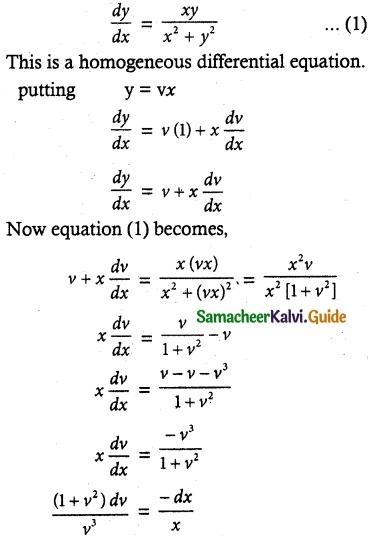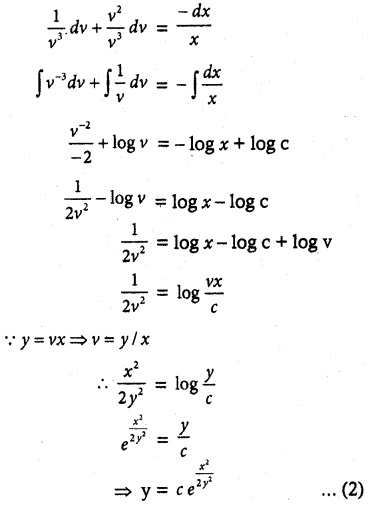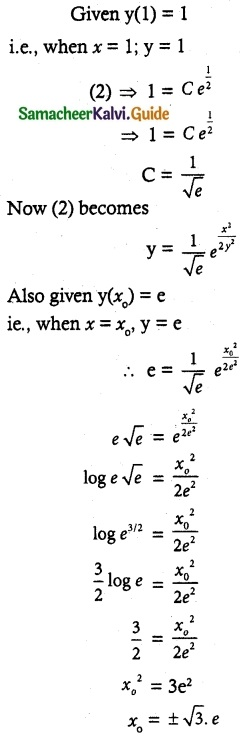Tamilnadu State Board New Syllabus Samacheer Kalvi 12th Maths Guide Pdf Chapter 10 Ordinary Differential Equations Ex 10.6 Textbook Questions and Answers, Notes.

## Tamilnadu Samacheer Kalvi 12th Maths Solutions Chapter 10 Ordinary Differential Equations Ex 10.6

Question 1.
Solve the following differential equations.
[x + y cos($$\frac { y }{ x }$$)] dx = x cos ($$\frac { y }{ x }$$) dy
Solution:
The given equation can be written as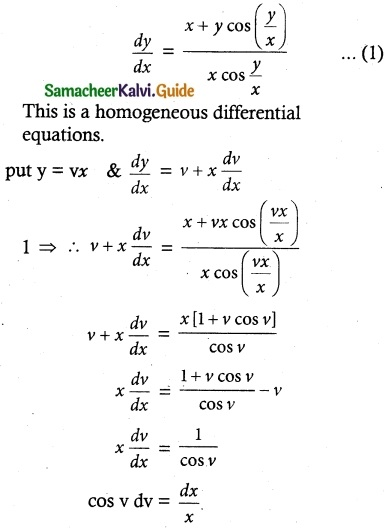On integration we obtain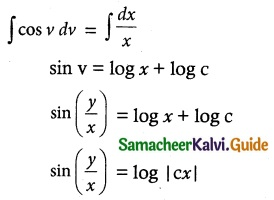which gives the required solution.Question 2.
Solve (x³ + y³)dy – x² ydx = 0
Solution:
The given equation can be written as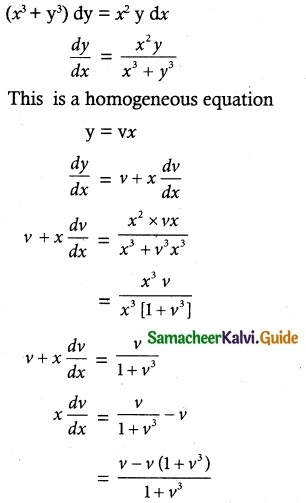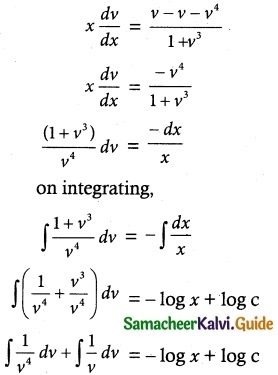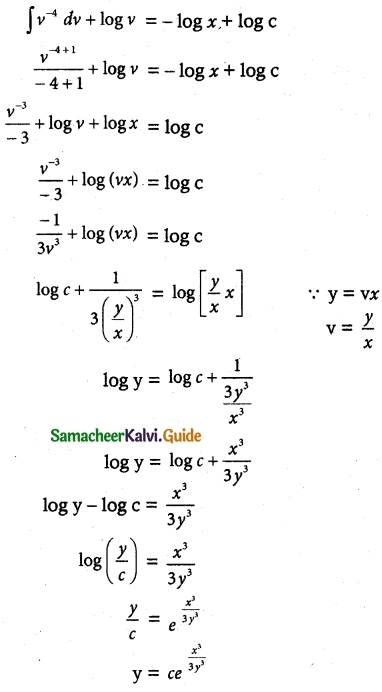Question 3.
Solve ye$$\frac { x }{ y }$$ dx = (x$$\frac { x }{ y }$$ + y)dy
Solution:
The given equation can be written as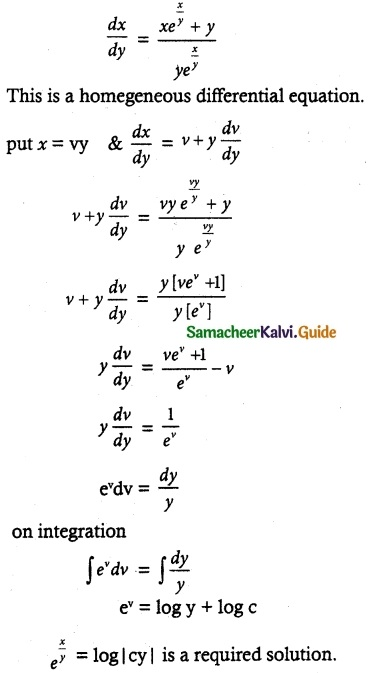Question 4.
Solve 2xy dx + (x² + 2y²)dy = 0
Solve ye$$\frac { x }{ y }$$ dx = (x$$\frac { x }{ y }$$ + y)dy
Solution:
The given differential equation can be written as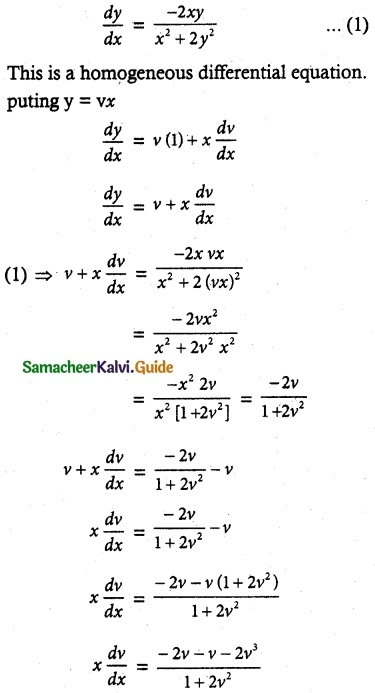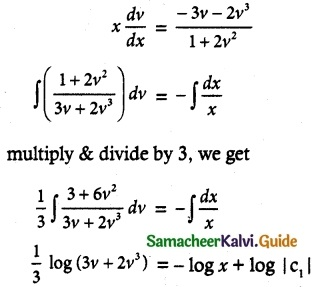$$\frac { 1 }{ 3 }$$ log (3v + 2v³) + log x = log |C1|
log (3v + 2v³) + 3log (x) = 3 log (C1)
log (3v + 2v³) + log (x)³ = log (C1
log (3v + 2v³)x³ = log C1³
(3v + 2v³)x³ = C1³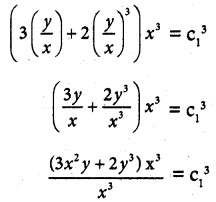3x²y + 2y³ = C1³
3x²y + 2y³ = C is a required solution.Question 5.
(y² – 2xy) dx = (x² – 2xy) dy
Solution:
Given equation is (y² – 2xy) dx = (x² – 2xy) dy
y² – 2xy = (x² – 2xy) $$\frac { dy }{ dx }$$
∴ The equation can written as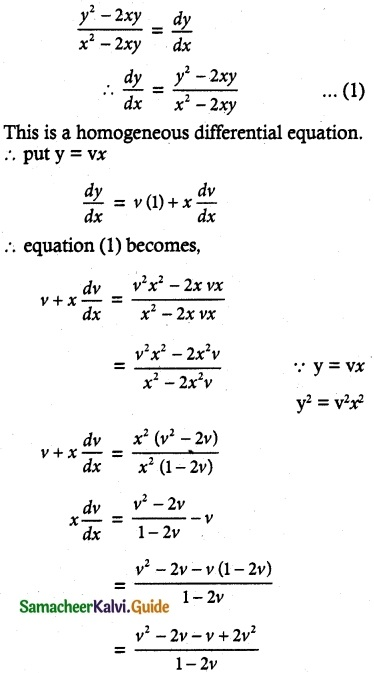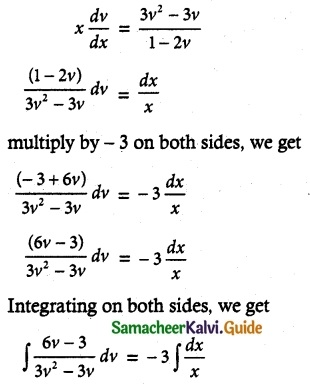log (3v² – 3v) = – 3 log x + log C
log (3v² – 3v) = – log x³ + log C
= log c – log x³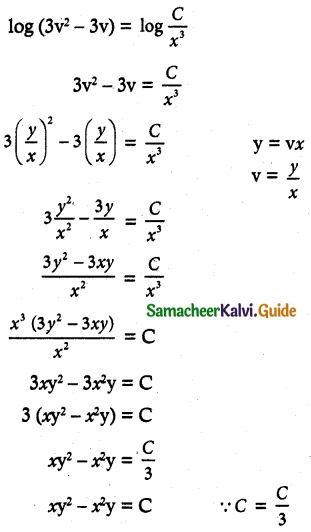Question 6.
x $$\frac { dy }{ dx }$$ = y – x cos²($$\frac { y }{ x }$$)
Solution:
Given x $$\frac { dy }{ dx }$$ = y – x cos² $$\frac { y }{ x }$$
The equation can be written as
$$\frac { dy }{ dx }$$ = $$\frac { y-cos^2 \frac { y }{ x } }{ x }$$ …….. (1)
This is a homogeneous differential equation.
y = vx
$$\frac { dy }{ dx }$$ = v (1) + x $$\frac { dv }{ dx }$$
Substituting $$\frac { dy }{ dx }$$ value in equation (1), we get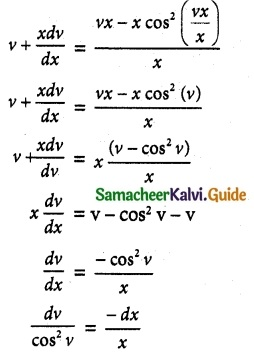Integrating on both sides, we get
∫ sec² v dx = -∫ $$\frac { dx }{ x }$$
tan v = – log x + log C
tan v = log C – log x
tan v = log($$\frac { C }{ x }$$)
etan v = $$\frac { C }{ x }$$
C = x etan v
C = x etan $$\frac { y }{ x }$$
Is a required equation.Question 7.
Solve (1 + 3e$$\frac { y }{ x }$$) dy + 3etan $$\frac { y }{ x }$$ (1 – $$\frac { y }{ x }$$) dx = 0, given that y = 0 when x = 1.
Solution:
The given differential equation may be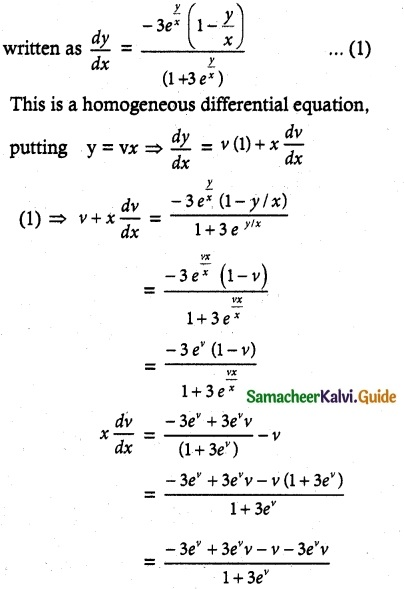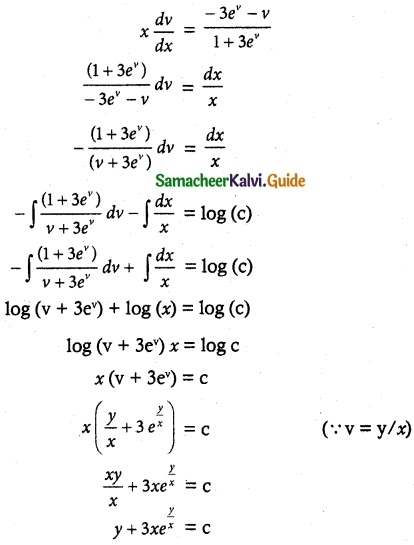Given that y = 0 when x = 1
0 + 3(1) e° = c
3 = c
∴ y + 3xey/x = 3 is a required solution.Question 8.
(x² + y²) dy = xy dx. It is given that y (1) = y(x0) = e. Find the value of x0.
Solution:
The given differential equation is of the form